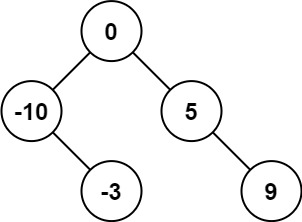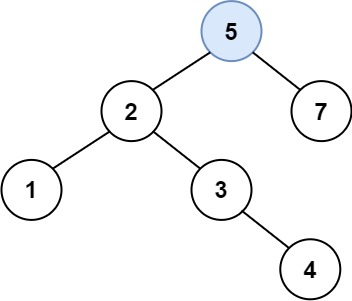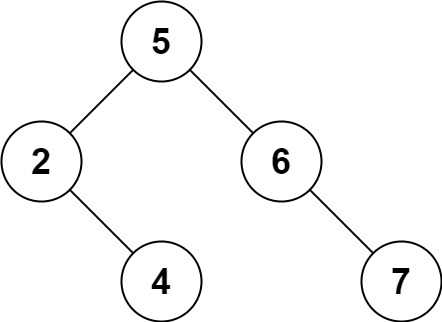# Posts published in “Binary Search Tree”

Given an integer array `nums` where the elements are sorted in ascending order, convert it to a height-balanced binary search tree.

height-balanced binary tree is a binary tree in which the depth of the two subtrees of every node never differs by more than one.

Example 1:

```Input: nums = [-10,-3,0,5,9]
Output: [0,-3,9,-10,null,5]
Explanation: [0,-10,5,null,-3,null,9] is also accepted:```

Example 2:

```Input: nums = [1,3]
Output: [3,1]
Explanation: [1,3] and [3,1] are both a height-balanced BSTs.
```

Constraints:

• `1 <= nums.length <= 104`
• `-104 <= nums[i] <= 104`
• `nums` is sorted in a strictly increasing order.

Idea:

Recursion + Divide and Conquer

Time complexity: O(n)
Space complexity: O(logn)

Solution:

``````/**
* Definition for a binary tree node.
* function TreeNode(val, left, right) {
*     this.val = (val===undefined ? 0 : val)
*     this.left = (left===undefined ? null : left)
*     this.right = (right===undefined ? null : right)
* }
*/
/**
* @param {number[]} nums
* @return {TreeNode}
*/
var sortedArrayToBST = function(nums) {
return createBST(nums, 0, nums.length - 1);
};

function createBST(nums, l, r) {
// exit recursion condition
if (l > r) return null;

let mid = l + Math.floor((r - l) / 2);
let root = new TreeNode(nums[mid]);
root.left = createBST(nums, l, mid - 1);
root.right = createBST(nums, mid + 1, r);
return root;
}``````

You are given the `root` of a binary search tree (BST), where exactly two nodes of the tree were swapped by mistake. Recover the tree without changing its structure.

Follow up: A solution using `O(n)` space is pretty straight forward. Could you devise a constant space solution?

Example 1:

```Input: root = [1,3,null,null,2]
Output: [3,1,null,null,2]
Explanation: 3 cannot be a left child of 1 because 3 > 1. Swapping 1 and 3 makes the BST valid.
```

Example 2:

```Input: root = [3,1,4,null,null,2]
Output: [2,1,4,null,null,3]
Explanation: 2 cannot be in the right subtree of 3 because 2 < 3. Swapping 2 and 3 makes the BST valid.
```

Constraints:

• The number of nodes in the tree is in the range `[2, 1000]`.
• `-231 <= Node.val <= 231 - 1`

Idea:

Because inorder traverse BST, all values are sorted. We can use inorder traversal to find two nodes that have prev.val > root. val and swap them

Time complexity: O(n)
Space complexity: O(height)

Solution:

``````/**
* Definition for a binary tree node.
* function TreeNode(val, left, right) {
*     this.val = (val===undefined ? 0 : val)
*     this.left = (left===undefined ? null : left)
*     this.right = (right===undefined ? null : right)
* }
*/
/**
* @param {TreeNode} root
* @return {void} Do not return anything, modify root in-place instead.
*/
var recoverTree = function(root) {
// javascript can't pass value by reference
// so pass array object by reference
let first = [null];
let second = [null];
let prev = [null];
inorder(root, first, second, prev);
[first.val, second.val] = [second.val, first.val];
};

function inorder(root, first, second, prev) {
// Because inorder traverse BST, all values are sorted.
// Using inorder traversal to find two nodes that have prev.val > root.val
if (root === null) return;
inorder(root.left, first, second, prev);
if (prev && prev.val > root.val) {
if (first === null) first = prev;
second = root;
}
prev = root;
inorder(root.right, first, second, prev);
}``````

Given the `root` of a binary search tree, and an integer `k`, return the `kth` (1-indexedsmallest element in the tree.

Example 1:

```Input: root = [3,1,4,null,2], k = 1
Output: 1
```

Example 2:

```Input: root = [5,3,6,2,4,null,null,1], k = 3
Output: 3
```

Constraints:

• The number of nodes in the tree is `n`.
• `1 <= k <= n <= 104`
• `0 <= Node.val <= 104`

Idea:

Recursive Inorder Traversal

Solution:

``````/**
* Definition for a binary tree node.
* function TreeNode(val, left, right) {
*     this.val = (val===undefined ? 0 : val)
*     this.left = (left===undefined ? null : left)
*     this.right = (right===undefined ? null : right)
* }
*/
/**
* @param {TreeNode} root
* @param {number} k
* @return {number}
*/
var kthSmallest = function(root, k) {
const nums = [];
inorder(root, nums);
return nums[k - 1];
};

function inorder(root, nums) {
if (root === null) return;
inorder(root.left, nums);
nums.push(root.val);
inorder(root.right, nums);
}``````

You are given the `root` node of a binary search tree (BST) and a `value` to insert into the tree. Return the root node of the BST after the insertion. It is guaranteed that the new value does not exist in the original BST.

Notice that there may exist multiple valid ways for the insertion, as long as the tree remains a BST after insertion. You can return any of them.

Example 1:

```Input: root = [4,2,7,1,3], val = 5
Output: [4,2,7,1,3,5]
Explanation: Another accepted tree is:```

Example 2:

```Input: root = [40,20,60,10,30,50,70], val = 25
Output: [40,20,60,10,30,50,70,null,null,25]
```

Example 3:

```Input: root = [4,2,7,1,3,null,null,null,null,null,null], val = 5
Output: [4,2,7,1,3,5]
```

Constraints:

• The number of nodes in the tree will be in the range `[0, 104]`.
• `-108 <= Node.val <= 108`
• All the values `Node.val` are unique.
• `-108 <= val <= 108`
• It’s guaranteed that `val` does not exist in the original BST.

Solution: (Recursion)

• Time complexity: O(logn ~ n)
• Space complexity: O(logn ~ n)
``````/**
* Definition for a binary tree node.
* function TreeNode(val, left, right) {
*     this.val = (val===undefined ? 0 : val)
*     this.left = (left===undefined ? null : left)
*     this.right = (right===undefined ? null : right)
* }
*/
/**
* @param {TreeNode} root
* @param {number} val
* @return {TreeNode}
*/
var insertIntoBST = function(root, val) {
// empty tree case:
if (root === null) return new TreeNode(val);
if (val > root.val) {
root.right = insertIntoBST(root.right, val);
} else {
root.left = insertIntoBST(root.left, val);
}
return root;
};``````

Given a root node reference of a BST and a key, delete the node with the given key in the BST. Return the root node reference (possibly updated) of the BST.

Basically, the deletion can be divided into two stages:

1. Search for a node to remove.
2. If the node is found, delete the node.

Follow up: Can you solve it with time complexity `O(height of tree)`?

Example 1:

```Input: root = [5,3,6,2,4,null,7], key = 3
Output: [5,4,6,2,null,null,7]
Explanation: Given key to delete is 3. So we find the node with value 3 and delete it.
One valid answer is [5,4,6,2,null,null,7], shown in the above BST.
Please notice that another valid answer is [5,2,6,null,4,null,7] and it's also accepted.```

Example 2:

```Input: root = [5,3,6,2,4,null,7], key = 0
Output: [5,3,6,2,4,null,7]
Explanation: The tree does not contain a node with value = 0.
```

Example 3:

```Input: root = [], key = 0
Output: []
```

Constraints:

• The number of nodes in the tree is in the range `[0, 104]`.
• `-105 <= Node.val <= 105`
• Each node has a unique value.
• `root` is a valid binary search tree.
• `-105 <= key <= 105`

Solution: (Recursion)

``````/**
* Definition for a binary tree node.
* function TreeNode(val, left, right) {
*     this.val = (val===undefined ? 0 : val)
*     this.left = (left===undefined ? null : left)
*     this.right = (right===undefined ? null : right)
* }
*/
/**
* @param {TreeNode} root
* @param {number} key
* @return {TreeNode}
*/
var deleteNode = function(root, key) {
if (root === null) return root;
if (key > root.val) {
root.right = deleteNode(root.right, key);
} else if (key < root.val) {
root.left = deleteNode(root.left, key);
} else { // found the key
// has children
if (root.left !== null && root.right !== null) {
let min = new TreeNode();
min = root.right;
while (min.left !== null) min = min.left;
root.val = min.val;
root.right = deleteNode(root.right, min.val);
} else { // no children
let newRoot = new TreeNode();
newRoot = root.left === null ? root.right : root.left;
root.left = root.right = null;
return newRoot;
}
}
return root;
};``````

Given the `root` of a binary tree, determine if it is a valid binary search tree (BST).

valid BST is defined as follows:

• The left subtree of a node contains only nodes with keys less than the node’s key.
• The right subtree of a node contains only nodes with keys greater than the node’s key.
• Both the left and right subtrees must also be binary search trees.

Example 1:

```Input: root = [2,1,3]
Output: true
```

Example 2:

```Input: root = [5,1,4,null,null,3,6]
Output: false
Explanation: The root node's value is 5 but its right child's value is 4.
```

Constraints:

• The number of nodes in the tree is in the range `[1, 104]`.
• `-231 <= Node.val <= 231 - 1`

Idea:

Use Binary Tree Inorder Traveral Template

Solution 1:

``````/**
* Definition for a binary tree node.
* function TreeNode(val, left, right) {
*     this.val = (val===undefined ? 0 : val)
*     this.left = (left===undefined ? null : left)
*     this.right = (right===undefined ? null : right)
* }
*/
/**
* @param {TreeNode} root
* @return {boolean}
*/
var isValidBST = function(root) {
// use inorder traversal template (2 whiles, 里左外右)
let preVal = -Infinity;
let stack = [];

while (root !== null || stack.length !== 0) {
while (root !== null) {
stack.push(root);
root = root.left;
}
root = stack.pop();
if (root.val <= preVal) return false;
preVal = root.val;
root = root.right;
}
return true;
};``````

Solution 2: (Recursion)

``````var isValidBST = function(root) {
return helper(root, null, null);
}

function helper(node, low, high) {
if (node === null) return true;
const val = node.val;
if ((low !== null && val <= low) || (high !== null && val >= high))
return false;
return helper(node.right, val, high) && helper(node.left, low, val);
}``````